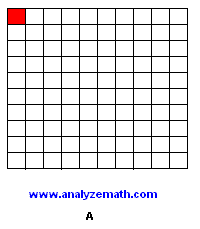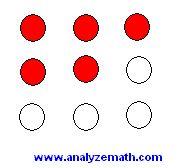Free Mathematics TutorialsPercent Maths Questions

Several questions on percentages, with detailed solutions are presented.

Question 1

What percentage of the large square is coloured red in each figure below?Solution to Question 1
In figure A, 1 small square out of a total of 100 is colored red, the answer is
1 / 100 = 1%
In figure B, 41 small squares out of a total of 100 are colored red, the answer is
41 / 100 = 41%
In figure C, 50 small squares out of a total of 100 are colored red, the answer is
50 / 100 = 50% which is also equal to one half.
In figure D, 100 small squares out of a total of 100 are colored red, the answer is
100 / 100 = 100% which is also equal to one.

Question 2

What percent of the balls in the figure below are red?Solution to Question 2
5 balls out of a total of nine are red, so in fraction we have
5 / 9
divide 5 by 9 to obtain
5 / 9 = 0.56 (rounded to 2 decimal places)
Multiply and divide 0.56 by 100 to obtain
= 0.56 * 100 / 100 = 56 / 100 = 56%
Approximately 56% of the balls in the above figure are red.

Question 3

What is 12% of 250?

Solution to Question 3
"what" in the above question is the unknown; we denote by y. The word "of" represents a multiplication and "is" represents equal. The above question may be translated in mathematical form as follows
y = 12% * 250
Rewrite the percentage ( 12% ) as a fraction in the above equation
y = [ 12 / 100 ] * 250 = [12 * 250 ] / 100 = 30
12% of 250 is equal to 30.

Question 4

4 is what percent of 32?

Solution to Question 4
Let the unknown "what percent" be denoted by y%, since we are looking for a percentage. "is" is represented by equal and "of" by a multiplication. Hence the above question may be translated into a math equation as follows:
4 = y% * 32
We now solve for the unknown y%
y% = 4 / 32 = 0.125
We have found the decimal form to y% which can be changed into percentage form by multiplying and dividing it by 100. Hence
y% = 0.125 = 12.5 / 100 = 12.5%
As an exercise, check that 12.5% of 32 gives 4.

Question 5

6 is 80% of what number?

Solution to Question 5
Let x be the unknown, "what number" in the above question. The word "of" represents a multiplication and "is" represent =. Hence the above question may be translated into a math equation as follows:
6 = 80% * x
Solve for x to obtain
x = 6 / 80%
Rewrite the percentage 80% as a fraction in the above equation.
x = 6 / [80 / 100]
To divide 6 by the fraction 80/100 multiply by the reciprocal of the fraction 80/100. Hence.
x = 6 * [100 / 80] = 600 / 80 = 7.5
As an exercise, check that 80% of 7.5 gives 6.

Question 6

A number increased from 120 to 150. By what percent, of its original value, did this number increase?
Solution to Question 6
A percent represent a part of a whole or a fraction with the denominator equal to 100. The part here is the absolute increase given by
part = 150 - 120 = 30
The whole is the original value, 120, hence the unknown percent x is given by
x = part / whole = 30 / 120 = 0.25
Multiply 0.25 by 100 and divide it by 100 to obtain.
x = 0.25 * 100 / 100 = 25 / 100 = 25%
An increase from 120 to 150 is equivalent to a 25% increase of the original value 120.

Question 7

A number decreased from 80 to 50. By what percent of its original value has this number decreased?
Solution to Question 7
The part here is given by
part = 80 - 50 = 30
The whole is the original value, 80, hence the percent x is given by
x = part / whole = 30 / 80 = 0.375
Multiply 0.375 by 100 and divide it by 100 to convert it into percentage.
x = 0.375* 100 / 100 = 37.5 / 100 = 37.5%
A decrease from 80 to 50 is equivalent to a 37.5% decrease of the original value 80.

Question 8

Which is greater 15% of 380 or 25 of 210?
Solution to Question 8
Let x = 15% of 380, hence
x = 15% * 380 = (15 / 100) * 80 = 15 * 380/100 = 57
Let y = 25% of 210, hence
y = 25% * 210 = (25/100) * 210 = 52.5
15% of 380 is greater than 25 of 210